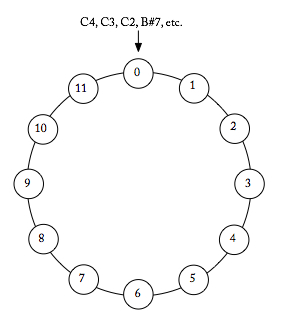What time is four hours later than 10 o’clock?

When we make calculations like this, we are doing modular arithmetic. Modular arithmetic is like regular arithmetic, except that the numbers “wrap around” or restart when they reach a certain value, called the modulus. In the case of our 12-hour clock, the modulus is 12.

Musical structures can often be best understood using this modular arithmetic. Think of the C-major scale. We begin on C, then D, E, F, G and back to A before B and returning to C. This is a modular system — we might call it modulo-G because after G we go back to the beginning (A).

In post-tonal music, once we assume octave and enharmonic equivalence, our pitch-class environment includes twelve unique pitch classes, just like the twelve hours on the clock. In this universe, modular arithmetic is a very useful way to imagine getting around.

Counting in this modulo 12 (or mod12) universe works just as in basic math [“1, 2, 3, …”], but after 11, we “begin again” at 0. (Note that while clocks start at 1 and end on 12, modular arithmetic always (re)starts with zero.) Conversely, when counting down [“10, 9, 8, …”], we follow 0 with 11.

While we are used to thinking of numbers on an infinite line, modular thinking wraps them into a finite number, generally represented by a circle. On this circle, all values are a number from 0 to 11.

Pitch-Class Space